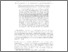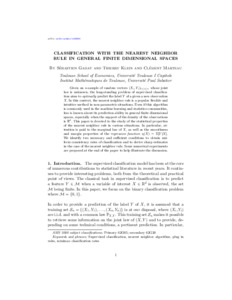Classification with the nearest neighbor rule in general finite dimensional spaces

Gadat, Sébastien, Klein, Thierry and Marteau, Clément (2016) Classification with the nearest neighbor rule in general finite dimensional spaces. Annals of Statistics, 44 (3). pp. 982-1009.

This is the latest version of this item.Preview
Text
Official URL: http://tse-fr.eu/pub/29830

Abstract

Given an n-sample of random vectors (Xi,Yi)1=i=n whose joint law is unknown, the long-standing problem of supervised classification aims to optimally predict the label Y of a given new observation X. In this context, the k-nearest neighbor rule is a popular flexible and intuitive method in nonparametric situations. Even if this algorithm is commonly used in the machine learning and statistics communities, less is known about its prediction ability in general finite dimensional spaces, especially when the support of the density of the observations is Rd . This paper is devoted to the study of the statistical properties of the k-nearest neighbor rule in various situations. In particular, attention is paid to the marginal law of X, as well as the smoothness and margin properties of the regression function n(X) = E[Y |X]. We identify two necessary and sufficient conditions to obtain uniform consistency rates of classification and derive sharp estimates in the case of the k-nearest neighbor rule. Some numerical experiments are proposed at the end of the paper to help illustrate the discussion

Item Type: Article English 2016 Yes Supervised classification, nearest neighbor algorithm, plug in rules, minimax classification rates B- ECONOMIE ET FINANCE TSE-R (Toulouse) UT1 05 Nov 2015 15:11 07 Mar 2018 13:23 oai:tse-fr.eu:29830 http://publications.ut-capitole.fr/id/eprint/18601

Available Versions of this ItemView Item